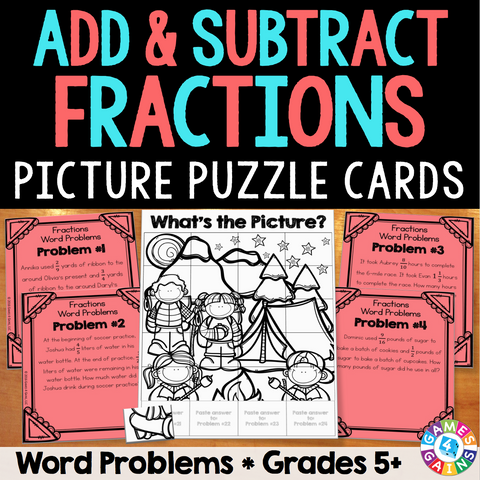Fraction Word Problems Picture Puzzle

• \$350

Fraction Word Problems Picture Puzzle Cards make solving addition and subtraction word problems fun! Included are 24 challenging one-step and multi-step word problem cards and a picture puzzle for students to put together as they work their way through each problem.

As the teacher, you will place the cards all around the room. Students will solve the fraction word problems on each card. Then, students will use their answers to put together the picture puzzle.

These cards support Common Core standard 5.NF.A.2: Solve word problems involving addition and subtraction of fractions referring to the same whole, including cases of unlike denominators.

IMPORTANT: These word problems include adding and subtracting both proper fractions and mixed numbers with unlike denominators. All answers must be simplified.

Included with this Fraction Word Problems Picture Puzzle Cards set:
• Instructions for use
• 24 fraction word problem cards
• "Picture Puzzle" sheet
• "What's the Picture?" sheet